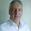# Before choosing courseCourse offering missing for current semester as well as for previous and coming semesters

## Content and learning outcomes

### Course contents

LINEAR ALGEBRA

• Complex numbers: The complex plane. Modulus and argument. Polar, rectangular and exponential form.
• Euler´s and de Moivre´s theorems. Binomial equations. Algebraic equations.
• Systems of linear equations.  Gauss elimination method.
• Vectors. Linear independent vectors
• Dot product, vector cross product, scalar triple product,
• Equations oflinesin 3D. Equations ofplanesin 3D
• Determinant. Matrices, matrix operations.
• Eigenvalues, eigenvectors.

CALCULUS

• Concepts of function, limits and continuity.
• Elementary functions.
• Differentiation rules.
• Application to graphing, rates, and extremum problems.
• L'Hospital's rule.
• Definite and indefinite integration. Techniques of integration.
• Applications of integration.
• Differential equations:  First order ordinary differential equations. Separable differential equations. Linear first-order  differential equations.
• Linear higher-order differential equations. Linear differential equations with constant coefficients. Applications.

### Intended learning outcomes

After completing this course, students should demonstrate competency in the following skills:

• Define and interpret the fundamental concepts of linear algebra and calculus : vector, dot product, cross product, triple product, line, plane, matrix, determinant, limit, continuity, derivative, integral.
• Do calculations with complex numbers  in polar, rectangular and exponential form
• Solve and geometrically interpret systems of linear equations.
• Use vector algebra to evaluate projections, distance, areas and volumes.
• Sketch the graph of a function using asymptotes, critical points, the derivative test for increasing/decreasing functions, and concavity.
• Apply differentiation to solve applied max/min problems.
• Use L'Hospital's rule to evaluate certain indefinite forms
• Evaluate integrals using techniques of integration, such as substitution, and integration by parts.
• Use the methods of integration to evaluate areas and volumes.
• Solve first order differential equation.
•  Solve second order linear differential equation with constant coefficient.
•  Apply differential equations to different technical fields
• Use suitable software for symbolic as well as numerical solving mathematical   problems and applications mentioned above.

### Course Disposition

No information inserted

## Literature and preparations

### Specific prerequisites

No information inserted

### Recommended prerequisites

No information inserted

### Equipment

No information inserted

### Literature

ENGINEERING MATHEMATICS , fourth (4TH) edition, Anthony Croft , R.Davison, M.Hargreaves , J. Flint, ISBN 9780273719779

## Examination and completion

If the course is discontinued, students may request to be examined during the following two academic years.

A, B, C, D, E, FX, F

### Examination

• LAB1 - Laboratory Work, 2,0 hp, betygsskala: P, F
• TEN1 - Examination, 4,0 hp, betygsskala: A, B, C, D, E, FX, F
• TEN2 - Examination, 4,0 hp, betygsskala: A, B, C, D, E, FX, F

Based on recommendation from KTH’s coordinator for disabilities, the examiner will decide how to adapt an examination for students with documented disability.

The examiner may apply another examination format when re-examining individual students.

Passed exams:
TEN1,  (4 Cr,  Linear algebra,  written exam), grading: A/B/C/D/E/Fx/F
TEN2,  ( 4 Cr,  Calculus, written exam), grading: A/B/C/D/E/Fx/F

Passed lab work
One of the following mathematical software packages will be used: Matlab, Mathematica or Maple.

### Opportunity to complete the requirements via supplementary examination

No information inserted

### Opportunity to raise an approved grade via renewed examination

No information inserted

### ExaminerArmin Halilovic

### Ethical approach

• All members of a group are responsible for the group's work.
• In any assessment, every student shall honestly disclose any help received and sources used.
• In an oral assessment, every student shall be able to present and answer questions about the entire assignment and solution.

## Further information

### Course web

Further information about the course can be found on the Course web at the link below. Information on the Course web will later be moved to this site.

Course web HF1008

### Offered by

CBH/Biomedical Engineering and Health Systems

Technology

First cycle